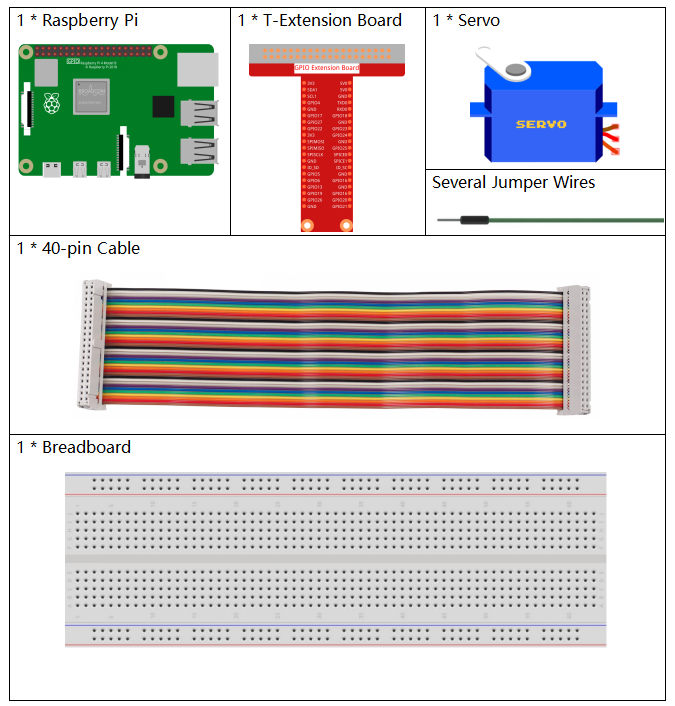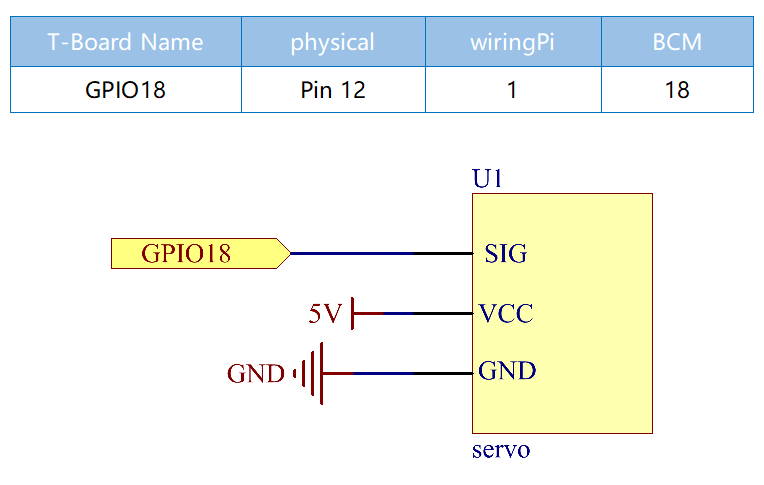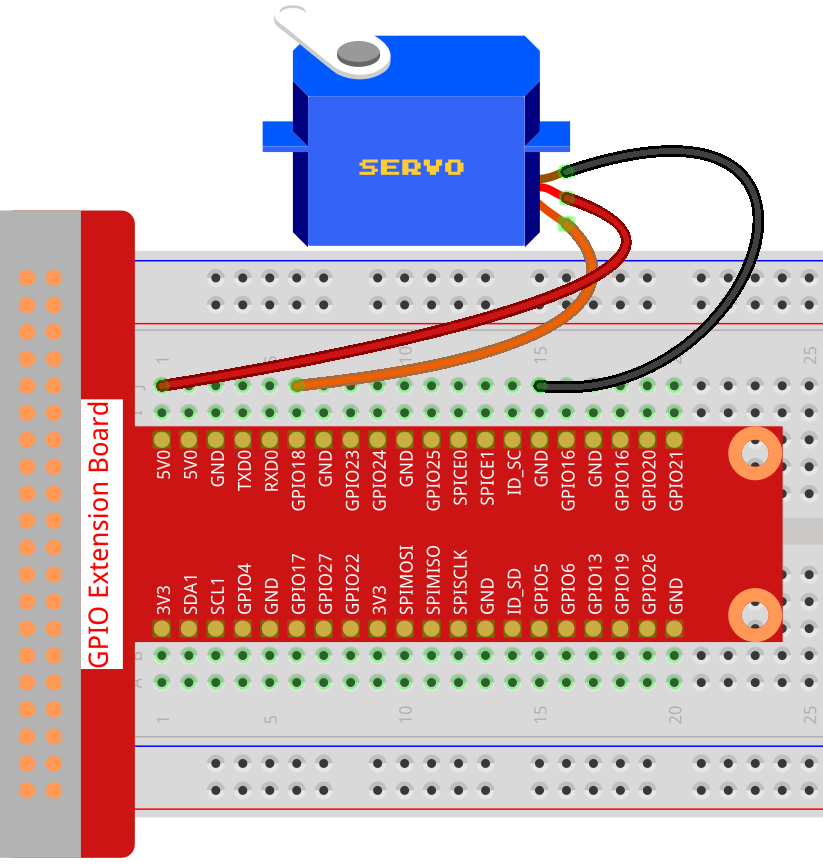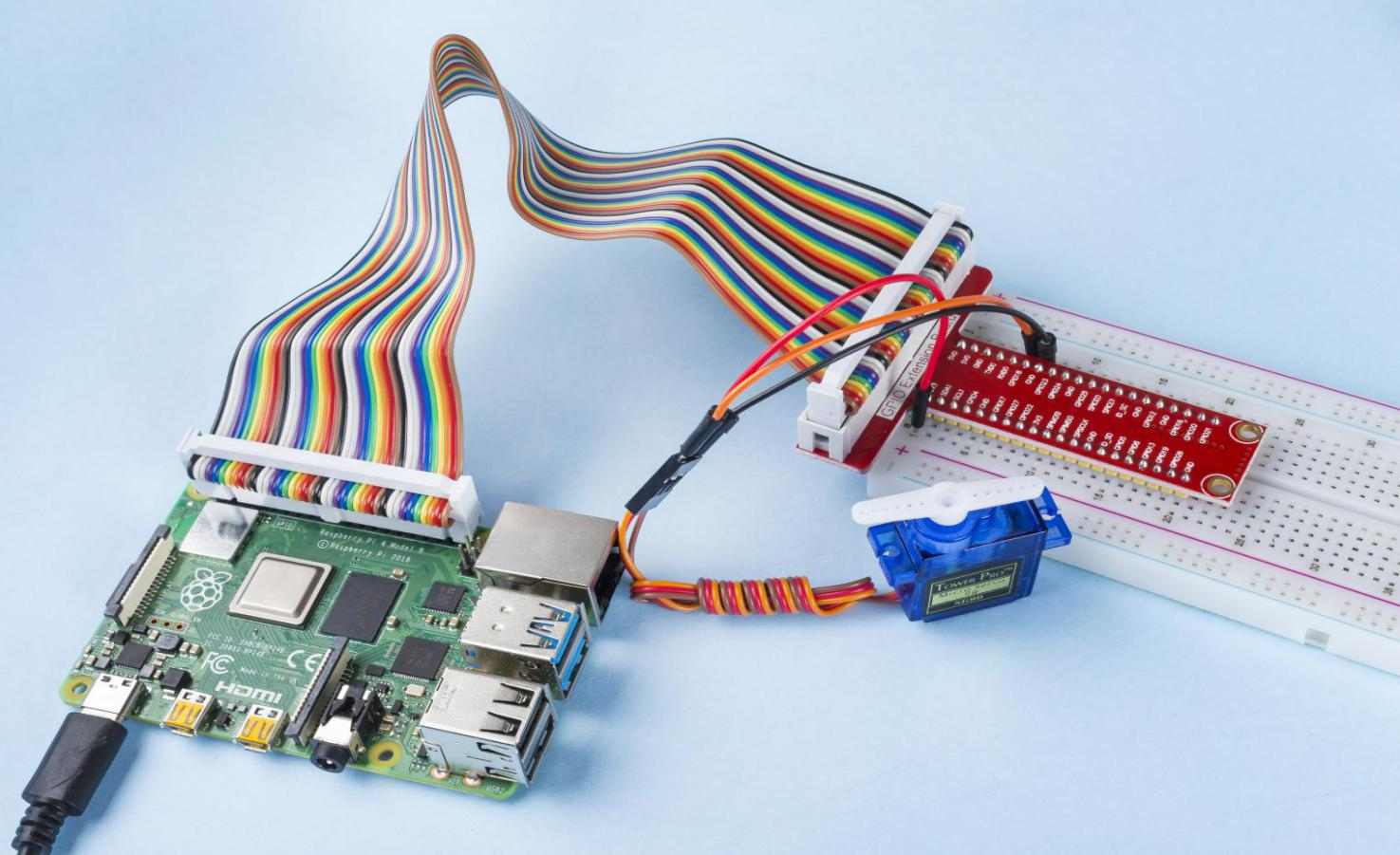# 1.3.2 Servo¶

## Introduction¶

In this project, we will learn how to make the servo rotate.

## Components¶## Schematic Diagram¶## Experimental Procedures¶

Step 1: Build the circuit.Step 2: Go to the folder of the code.

```cd /home/pi/raphael-kit/python/
```

Step 3: Run the executable file.

```sudo python3 1.3.2_Servo.py
```

After the program is executed, the servo will rotate from 0 degrees to 180 degrees, and then from 180 degrees to 0 degrees, circularly.

Code

Note

You can Modify/Reset/Copy/Run/Stop the code below. But before that, you need to go to source code path like `raphael-kit/python`. After modifying the code, you can run it directly to see the effect.

```import RPi.GPIO as GPIO
import time

SERVO_MIN_PULSE = 500
SERVO_MAX_PULSE = 2500
ServoPin = 18

def map(value, inMin, inMax, outMin, outMax):
return (outMax - outMin) * (value - inMin) / (inMax - inMin) + outMin

def setup():
global p
GPIO.setmode(GPIO.BCM)       # Numbers GPIOs by BCM
GPIO.setup(ServoPin, GPIO.OUT)   # Set ServoPin's mode is output
GPIO.output(ServoPin, GPIO.LOW)  # Set ServoPin to low
p = GPIO.PWM(ServoPin, 50)     # set Frequecy to 50Hz
p.start(0)                     # Duty Cycle = 0

def setAngle(angle):      # make the servo rotate to specific angle (0-180 degrees)
angle = max(0, min(180, angle))
pulse_width = map(angle, 0, 180, SERVO_MIN_PULSE, SERVO_MAX_PULSE)
pwm = map(pulse_width, 0, 20000, 0, 100)
p.ChangeDutyCycle(pwm)#map the angle to duty cycle and output it
def loop():
while True:
for i in range(0, 181, 5):   #make servo rotate from 0 to 180 deg
setAngle(i)     # Write to servo
time.sleep(0.002)
time.sleep(1)
for i in range(180, -1, -5): #make servo rotate from 180 to 0 deg
setAngle(i)
time.sleep(0.001)
time.sleep(1)
def destroy():
p.stop()
GPIO.cleanup()

if __name__ == '__main__':     #Program start from here
setup()
try:
loop()
except KeyboardInterrupt:  # When 'Ctrl+C' is pressed, the program destroy() will be executed.
destroy()
```

Code Explanation

```p = GPIO.PWM(ServoPin, 50)     # set Frequecy to 50Hz
p.start(0)                     # Duty Cycle = 0
```

Set the servoPin to PWM pin, then the frequency to 50hz, and the period to 20ms.

p.start(0): Run the PWM function，and set the initial value to 0.

```def setAngle(angle):      # make the servo rotate to specific angle (0-180 degrees)
angle = max(0, min(180, angle))
pulse_width = map(angle, 0, 180, SERVO_MIN_PULSE, SERVO_MAX_PULSE)
pwm = map(pulse_width, 0, 20000, 0, 100)
p.ChangeDutyCycle(pwm)#map the angle to duty cycle and output it
```

Create a function, setAngle() to write angle that ranges from 0 to 180 into the servo.

```angle = max(0, min(180, angle))
```

This code is used to limit the angle within the range 0-180°.

The min() function returns the minimum of the input values. If 180<angle, then return 180,if not, return angle.

The max() method returns the maximum element in an iterable or largest of two or more parameters. If 0>angle, then return 0, if not, return angle.

```pulse_width = map(angle, 0, 180, SERVO_MIN_PULSE, SERVO_MAX_PULSE)
pwm = map(pulse_width, 0, 20000, 0, 100)
p.ChangeDutyCycle(pwm)
```

To render a range 0 ~ 180 ° to the servo, the pulse width of the servo is set to 0.5ms(500us)-2.5ms(2500us).

The period of PWM is 20ms(20000us), thus the duty cycle of PWM is (500/20000)%-(2500/20000)%, and the range 0 ~ 180 is mapped to 2.5 ~ 12.5.

## Phenomenon Picture¶